# Stability of a computational process

(diff) ← Older revision | Latest revision (diff) | Newer revision → (diff)

A property characterizing the speed of accumulation of gross computing errors. The concept of stability of a computational process was introduced because in real calculations one cannot operate with exact numbers and it is impossible to circumvent rounding, which is sometimes the cause of a fast loss of accuracy.

A computational process is a sequence of arithmetic operations on numbers. Let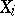be a normed linear space anda continuous operator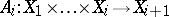. Then the sequence of equations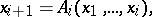(1)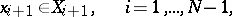gives a computational process with original data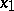and intermediate results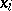,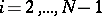. Usually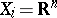and the operatorconsists of a finite number of arithmetic operations. As a rule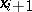depends not on all the intermediate results obtained earlier. The numbermay be given in advance or determined in the course of the computational process itself. In the latter casedepends on(e.g. ifis the number of iterations needed for a given degree of accuracy).

An actual computational process may not be carried out exactly in conformity with definition (1), because in carrying out the arithmetic operations rounding errors are introduced andmay be obtained from inexact previous results. This means that instead ofone has actually calculated the element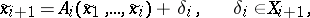(2)

where the small additive error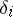arises by rounding in the course of applying the operator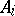. The value of, determined from the values of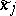,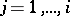, depends on the method of rounding, the working of the machine program, etc. However, even if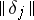is small for, this by itself will not guarantee that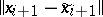is small. This difference will be small only for a so-called stable computational process, for which it is not strongly dependent on.

How to Cite This Entry:
Stability of a computational process. Encyclopedia of Mathematics. URL: http://encyclopediaofmath.org/index.php?title=Stability_of_a_computational_process&oldid=16612
This article was adapted from an original article by A.F. Shapkin (originator), which appeared in Encyclopedia of Mathematics - ISBN 1402006098. See original article